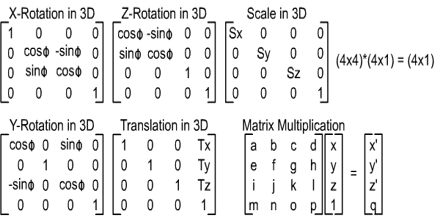Mathematic

# Matrix TransformationGeneral purpose of this lecture is to present on Matrix Transformation. A set of numbers arranged in rows and columns enclosed in round or square brackets is called a matrix. The order of a matrix gives the number of rows followed by the number of columns in a matrix. A matrix with an equal number of rows and columns is called a square matrix. A diagonal matrix has all its elements zero except for those in the leading diagonal (from top to bottom right). Two matrices are equal if, and only if, they are identical. This means they must be of the same order and the respective elements must be identical.